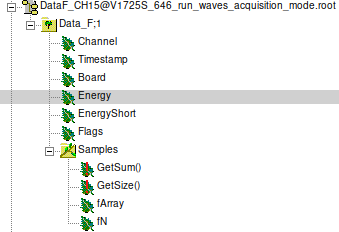# Unable to read a TTree with leaves and a branch (TArrayS) with pyROOT

Hello everyone, (first post here)

I am new to ROOT, and I am trying to read a .root using pyROOT. The .root comes from a Nuclear detector (CAEN).The root Tree looks like (I can not upload the .root because it exceeds the maximum size, it weights 6.8MB while the max size is 3.0MB):I am interested in the leaf ‘Energy’ and in the leaf “fArray”, which is inside the branch “Samples” and contains the waveform of the event. So, for each event, I have 1 value for Energy, Board, etc, but for the “Samples” I should have an array, creating the waveform of the event.

I am able to read the leaves within the Tree (Data_F) (Energy, Flags, etc), by doing (I am not using the AsMatrix function because it seems like it will be removed from ROOT soon):

``````data_root = TFile("DataF_CH15@V1725S_646_run_waves_acquisition_mode.root")
Tree=data_root.Data_F                         #getting the Tree
N = Tree.GetEntries()            #number of entries of the Tree (numbers)

E = np.array( [] )                        #store the Energy
Board = np.array( [] )                    #store the Board
Ch_digi = np.array( [] )                  #store the Channel
Timestamp = np.array( [] )                #store the Timestamp

for event in Tree:              #for each event, store the desired values
E = np.append(E, Tree.Energy)
Board = np.append(Board, Tree.Board)
Ch_digi = np.append(Ch_digi, Tree.Board)
Timestamp = np.append(Timestamp, Tree.Timestamp)
``````

But I can not do the same for the leave “fArray” inside the Branch “Samples”, because

Tree.Samples.fArray

is not a number (which was the case for the previous leaves), but a “<cppyy.LowLevelView at 0x7fb223e6c730>”, which is what python’s command line returns.

Another idea I got was to read the TTre using the Get functions:

``````Sample = Tree.GetBranch('Samples')          #the branch inside the root file
fArray = Sample.GetLeaf('fArray')           #the leaf inside the branch
#that contains the waveform
``````

But I do not know how could I get the data from that variable neither. Any help will be very appretiated. As a final comment, I think that Samples is a TArrayS, since if I type

Tree.Samples

I get:

<cppyy.gbl.TArrayS object at 0x55d0dbdd7040>

Dani

ROOT Version: 6.24/00
_Python Version: 3.8.5
_Using Spyder (python): 5.0.3

Hi,

You can probably iterate over the elements in that `Samples` array, with:

``````for event in Tree:
for elem in event.Samples.fArray:
print(elem) # this should print a number
``````
1 Like

Also, it looks like `fN` stores the length of the array, so you could do `event.Samples.fArray[i]` where `0 <= i < event.Samples.fN`

1 Like

Hello etejedor,

I deeply appreciate your fast answers, it turns out that both works. Furthermore, fN stores the length of the array.

I have chosen the second option (loop using event.Samples.farray[i]) because it is less demanding (I only need to use 1 event). Thank you so much